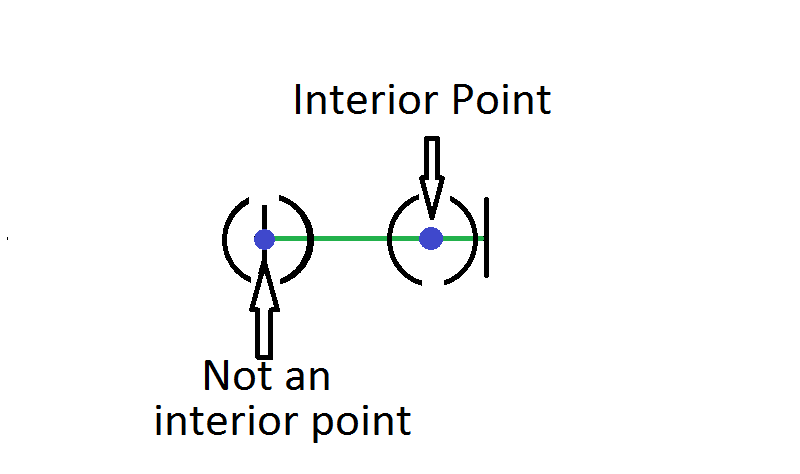Geometry

# Analysis Warmup

Which of these is the set of all interior points of the open interval $$(0,1)?$$

Definition. An interior point of a set $$S$$ of real numbers is a number $$x$$ such that the open interval $$(x - \epsilon, x + \epsilon)$$ is contained in $$S$$ for some positive number $$\epsilon.$$

Which of these is the set of all boundary points of the closed interval $$[0,1]$$?

Definition. A boundary point of a set $$S$$ of real numbers is a number $$x$$ such that every open interval $$(a, b)$$ contaning $$x$$ contains a number in $$S$$ and a number not in $$S.$$

Which of these is the set of all interior points of the closed interval $$[0,1]?$$

Definition. An interior point of a set $$S$$ of real numbers is a number $$x$$ such that the open interval $$(x - \epsilon, x + \epsilon)$$ is contained in $$S$$ for some positive number $$\epsilon.$$True or False?

The set of all boundary points (using the definition below) of the natural numbers $$\mathbb{N}$$ is $$\mathbb{N}.$$

Definition. A boundary point of a set $$S$$ of real numbers is a number $$x$$ such that every open interval $$(a, b)$$ containing $$x$$ contains a number in $$S$$ and a number not in $$S.$$

What is

$\bigcup_{n=3}^\infty \left[\frac{1}{n},1 - \frac{1}{n}\right]?$

Notation. The bracket notation represents a closed interval, so $$[3, 5] = \{x | 3 \leq x \leq 5\}$$ is the set of all real numbers greater than or equal to 3 and less than or equal to 5.

The $$\bigcup$$ symbol represents set union, so $$\{1, 2, 3\}\bigcup \{2,3,4\} = \{1, 2, 3, 4\}.$$ The indexing $$\bigcup_{n=3}^\infty$$ represents an union of infinitely many sets, i.e.

$\bigcup_{n=3}^\infty \left[\frac{1}{n},1 - \frac{1}{n}\right] = \left[\frac{1}{3},1-\frac{1}{3}\right] \bigcup \left[\frac{1}{4},1- \frac{1}{4}\right] \bigcup \left[\frac{1}{5},1- \frac{1}{5}\right] \bigcup \cdots$

×

Problem Loading...

Note Loading...

Set Loading...